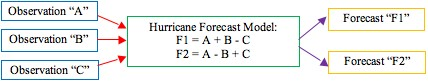How Hurricane Forecast Models WorkHurricane forecast models use observational data to describe the current state of the atmosphere and then solve the model’s mathematical equations to produce one or more forecasts. The observations are the input variables and the forecasts are the output variables. Consider this extremely simplified example that illustrates how the process works by using two equations to produce two forecasts (F1 and F2, where perhaps F1 is hurricane track and F2 is hurricane intensity) from three observations (A, B, and C):In this simple example, Forecast F1 and F2 are different because they are computing differing variables. In reality, hurricane forecast models are significantly more complex than is shown here, but the concept is the same.

The details that differ among various hurricane forecast models include:

• Which observations are fed into the model (set of blue boxes shown above)
• How the observations are read (assimilated) into the model (set of red arrows)
• What equations are solved by the model, and how they are solved (green box)
• How the solved equations are translated into useable forecasts (set of purple arrows)
• Which forecasts are obtained from the model (set of yellow boxes)

References